#Interactive Real Analysis

Next | Previous | Glossary | Map

## 3. Sequences of Numbers

### 3.1. Sequences

So far we have introduced sets as well as the number systems that we will use in this text. Next, we will study sequences of numbers. Sequences are, basically, countably many numbers arranged in an order that may or may not exhibit certain patterns. Here is the formal definition of a sequence:
 Definition 3.3.1: Sequence A sequence of real numbers is a function f: N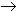R. In other words, a sequence can be written as f(1), f(2), f(3), ..... Usually, we will denote such a sequence by the symbol, where aj = f(j).
For example, the sequence 1, 1/2, 1/3, 1/4, 1/5, ... is written as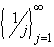. Keep in mind that despite the strange notation, a sequence can be thought of as an ordinary function. In many cases that may not be the most expedient way to look at the situation. It is often easier to simply look at a sequence as a 'list' of numbers that may or may not exhibit a certain pattern.

We now want to describe what the long-term behavior, or pattern, of a sequence is, if any.

 Definition 3.1.2: Convergence A sequenceof real (or complex) numbers is said to converge to a real (or complex) number c if for every> 0 there is an integer N > 0 such that if j > N then | aj - c | <The number c is called the limit of the sequenceand we sometimes write ajc. If a sequencedoes not converge, then we say that it diverges.
 Example 3.1.3:Consider the sequence. It converges to zero. Prove it.The sequence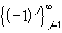does not converge. Prove it.The sequenceconverges to zero Prove it.
Convergent sequences, in other words, exhibit the behavior that they get closer and closer to a particular number. Note, however, that divergent sequence can also have a regular pattern, as in the second example above. But it is convergent sequences that will be particularly useful to us right now.

We are going to establish several properties of convergent sequences, most of which are probably familiar to you. Many proofs will use an 'argument' as in the proof of the next result. This type of argument is not easy to get used to, but it will appear again and again, so that you should try to get as familiar with it as you can.

 Proposition 3.1.4: Convergent Sequences are Bounded Letbe a convergent sequence. Then the sequence is bounded, and the limit is unique.

 Example 3.1.5:The Fibonacci numbers are recursively defined as x1 = 1, x2 = 1, and for all n > 2 we set xn = xn - 1 + xn - 2. Show that the sequence of Fibonacci numbers {1, 1, 2, 3, 5, ...} does not converge.
Convergent sequences can be manipulated on a term by term basis, just as one would expect:
 Proposition 3.1.6: Algebra on Convergent Sequences Supposeandare converging to a and b, respectively. Then Their sum is convergent to a + b, and the sequences can be added term by term. Their product is convergent to a * b, and the sequences can be multiplied term by term. Their quotient is convergent to a / b, provide that b # 0, and the sequences can be divided term by term (if the denominators are not zero). If anbn for all n, then ab

This theorem states exactly what you would expect to be true. The proof of it employs the standard trick of 'adding zero' and using the triangle inequality. Try to prove it on your own before looking it up.

Note that the fourth statement is no longer true for strict inequalities. In other words, there are convergent sequences with an < bn for all n, but strict inequality is no longer true for their limits. Can you find an example ?

While we now know how to deal with convergent sequences, we still need an easy criteria that will tell us whether a sequence converges. The next proposition gives reasonable easy conditions, but will not tell us the actual limit of the convergent sequence.

First, recall the following definitions:

 Definition 3.1.7: Monotonicity A sequenceis called monotone increasing if aj + 1aj for all j. A sequenceis called monotone decreasing if ajaj + 1 for all j.
In other words, if every next member of a sequence is larger than the previous one, the sequence is growing, or monotone increasing. If the next element is smaller than each previous one, the sequence is decreasing. While this condition is easy to understand, there are equivalent conditions that are often easier to check:
• Monotone increasing:
1. aj + 1aj
2. aj + 1 - aj0
3. aj + 1 / aj1, if aj > 0
• Monotone decreasing:
1. aj + 1aj
2. aj + 1 - aj0
3. aj + 1 / aj1, if aj > 0
 Examples 3.1.8:Is the sequence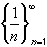monotone increasing or decreasing ?Is the sequence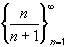monotone increasing or decreasing ?Is it true that a bounded sequence converges ? How about monotone increasing sequences ?
Here is a very useful theorem to establish convergence of a given sequence (without, however, revealing the limit of the sequence): First, we have to apply our concepts of supremum and infimum to sequences:

• If a sequenceis bounded above, then c = sup(xk) is finite. Moreover, given any> 0, there exists at least one integer k such that xk > c -, as illustrated in the picture.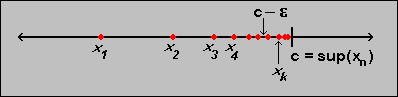• If a sequenceis bounded below, then c = inf(xk) is finite. Moreover, given any> 0, there exists at least one integer k such that xk < c +, as illustrated in the picture.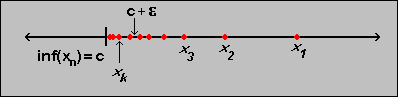Proposition 3.1.9: Monotone Sequences Ifis a monotone increasing sequence that is bounded above, then the sequence must converge.Ifis a monotone decreasing sequence that is bounded below, then the sequence must converge.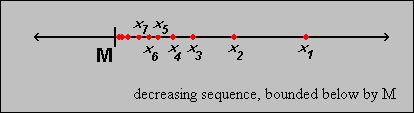Using this result it is often easy to prove convergence of a sequence just by showing that it is bounded and monotone. The downside is that this method will not reveal the actual limit, just prove that there is one.

 Examples 3.1.10:Prove that the sequencesandconverge. What is their limit?Define x1 = b and let xn = xn - 1 / 2 for all n > 1. Prove that this sequence converges for any number b. What is the limit ?Let a > 0 and x0 > 0 and define the recursive sequence xn+1 = 1/2 (xn + a / xn) Show that this sequence converges to the square root of a regardless of the starting point x0 > 0.
There is one more simple but useful theorem that can be used to find a limit if comparable limits are known. The theorem states that if a sequence is pinched in between two convergent sequences that converge to the same limit, then the sequence in between must also converge to the same limit.
 Theorem 3.1.11: The Pinching Theorem Suppose {aj} and {cj} are two convergent sequences such that lim aj = lim cj = L. If a sequence {bj} has the property that ajbjcj for all j, then the sequence {bj} converges and lim bj = L.
 Example 3.1.12:Show that the sequence sin(n) / n and cos(n) / n both converge to zero.
Next | Previous | Glossary | Map